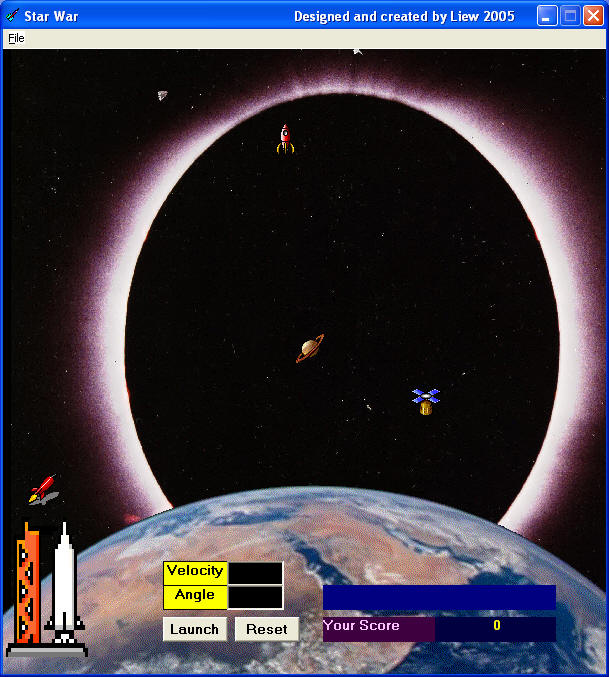# Star War

We have designed this game for educational purpose. This program demonstrates the principle of projectile in physics. At a certain angle and a certain launch velocity, the projectile can reach a certain range and certain height. The maximum range is at an angle of 45 degree. This principle can be applied in the military field where a missile can be launched at a certain velocity and certain angle to hit a remote target. It can also be applied in other scientific and technological fields. This game provides a good training for students in their abilities in making estimation.

In this program, we use the formulae v sin A - (1/2)gt2  as  the vertical component of the displacement and v cos A as the horizontal component  of the displacement( where g is the gravitational acceleration , v the launch velocity and A the launch angle). To enable the missile to fly, we used a combination of the Object.Move method  and the object coordinate system , i.e. object. left and object.Top.

We also use the randomize method so that the objects will appear at different positions randomly at each new game. In addition, we use the randomize method to load different backgrounds at start up and at each new game.

#### The Runtime Interface### The Code

'To randomly set the initial positions of the objects

left1 = Int(Rnd * 7000) + 1000
left2 = Int(Rnd * 7000) + 1000
left3 = Int(Rnd * 7000) + 1000
top1 = Int(Rnd * 5000) + 100
top2 = Int(Rnd * 5000) + 100
top3 = Int(Rnd * 5000) + 100
Image2.Left = left1
Image3.Left = left2
Image4.Left = left3
Image2.Top = top1
Image3.Top = top2
Image4.Top = top3
w = 0
score = 0
Label7.Caption = Str\$(score)
End Sub

Private Sub Image7_Click()
Label5.Visible = False
End Sub

Private Sub Timer1_Timer()

MMControl1.Command = "close"

If Image1.Left < 15000 And Image1.Top < 9000 Then

v = Val(Text1.Text)
a = Val(Text2.Text)
t = t + 1

' To use the formula vertical displacement=vsina- (1/2)gt^2 and horizontal displacement=vcosa*t
'So that it follows a parabolic trajectory

y = v * Sin(a * 3.141592654 / 180) * t - 4.9 * (t ^ 2)
x = v * Cos(a * 3.141592654 / 180) * t
Image1.Move Image1.Left + x, Image1.Top - y
If Image4.Visible = True And (Image1.Left < left3 + 240 And Image1.Left > left3 - 240) And (Image1.Top < top3 + 240 And Image1.Top > top3 - 240) Then
i = 2

Get the Starwar Complete